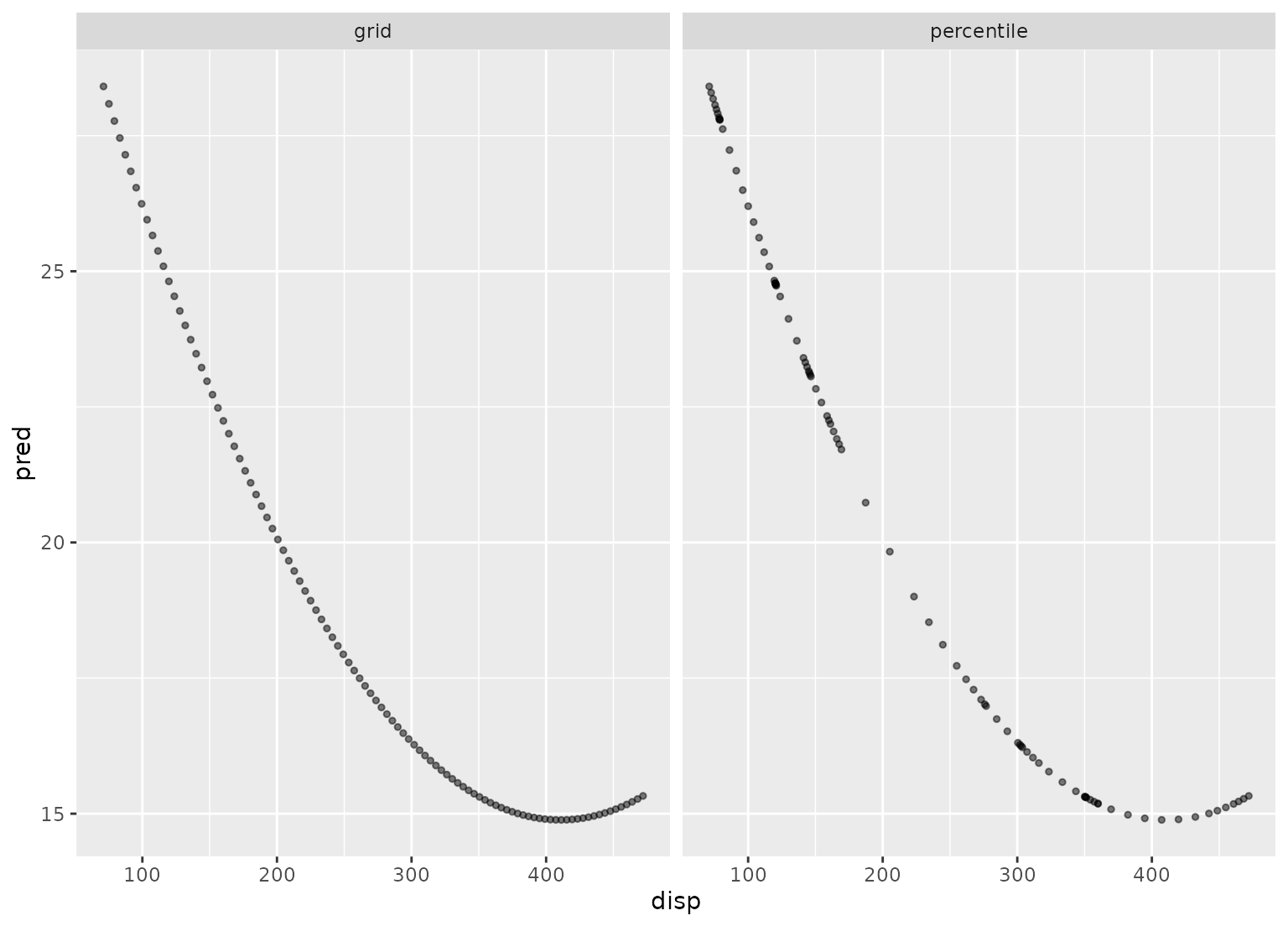step_profile() creates a specification of a recipe step that will fix the levels of all variables but one and will create a sequence of values for the remaining variable. This step can be helpful when creating partial regression plots for additive models.

## Usage

step_profile(
recipe,
...,
profile = NULL,
pct = 0.5,
index = 1,
grid = list(pctl = TRUE, len = 100),
columns = NULL,
role = NA,
trained = FALSE,
skip = FALSE,
id = rand_id("profile")
)

## Arguments

recipe

A recipe object. The step will be added to the sequence of operations for this recipe.

...

One or more selector functions to choose variables for this step. See selections() for more details.

profile

A call to dplyr::vars()) to specify which variable will be profiled (see selections()). If a column is included in both lists to be fixed and to be profiled, an error is thrown.

pct

A value between 0 and 1 that is the percentile to fix continuous variables. This is applied to all continuous variables captured by the selectors. For date variables, either the minimum, median, or maximum used based on their distance to pct.

index

The level that qualitative variables will be fixed. If the variables are character (not factors), this will be the index of the sorted unique values. This is applied to all qualitative variables captured by the selectors.

grid

A named list with elements pctl (a logical) and len (an integer). If pctl = TRUE, then len denotes how many percentiles to use to create the profiling grid. This creates a grid between 0 and 1 and the profile is determined by the percentiles of the data. For example, if pctl = TRUE and len = 3, the profile would contain the minimum, median, and maximum values. If pctl = FALSE, it defines how many grid points between the minimum and maximum values should be created. This parameter is ignored for qualitative variables (since all of their possible levels are profiled). In the case of date variables, pctl = FALSE will always be used since there is no quantile method for dates.

columns

A character string of the selected variable names. This field is a placeholder and will be populated once prep() is used.

role

Not used by this step since no new variables are created.

trained

A logical to indicate if the quantities for preprocessing have been estimated.

skip

A logical. Should the step be skipped when the recipe is baked by bake()? While all operations are baked when prep() is run, some operations may not be able to be conducted on new data (e.g. processing the outcome variable(s)). Care should be taken when using skip = TRUE as it may affect the computations for subsequent operations.

id

A character string that is unique to this step to identify it.

## Value

An updated version of recipe with the new step added to the sequence of any existing operations.

## Details

This step is atypical in that, when baked, the new_data argument is ignored; the resulting data set is based on the fixed and profiled variable's information.

## Tidying

When you tidy() this step, a tibble with columns terms (which is the columns that will be affected) and type (fixed or profiled) is returned.

## Case weights

The underlying operation does not allow for case weights.

## Examples

data(Sacramento, package = "modeldata")

# Setup a grid across beds but keep the other values fixed
recipe(~ city + price + beds, data = Sacramento) %>%
step_profile(-beds, profile = vars(beds)) %>%
prep(training = Sacramento) %>%
bake(new_data = NULL)
#> # A tibble: 6 × 3
#>   city      price  beds
#>   <fct>     <int> <int>
#> 1 ANTELOPE 220000     1
#> 2 ANTELOPE 220000     2
#> 3 ANTELOPE 220000     3
#> 4 ANTELOPE 220000     4
#> 5 ANTELOPE 220000     5
#> 6 ANTELOPE 220000     8

##########

# An *additive* model; not for use when there are interactions or
# other functional relationships between predictors

lin_mod <- lm(mpg ~ poly(disp, 2) + cyl + hp, data = mtcars)

# Show the difference in the two grid creation methods

disp_pctl <- recipe(~ disp + cyl + hp, data = mtcars) %>%
step_profile(-disp, profile = vars(disp)) %>%
prep(training = mtcars)

disp_grid <- recipe(~ disp + cyl + hp, data = mtcars) %>%
step_profile(
-disp,
profile = vars(disp),
grid = list(pctl = FALSE, len = 100)
) %>%
prep(training = mtcars)

grid_data <- bake(disp_grid, new_data = NULL)
grid_data <- grid_data %>%
mutate(
pred = predict(lin_mod, grid_data),
method = "grid"
)

pctl_data <- bake(disp_pctl, new_data = NULL)
pctl_data <- pctl_data %>%
mutate(
pred = predict(lin_mod, pctl_data),
method = "percentile"
)

plot_data <- bind_rows(grid_data, pctl_data)

library(ggplot2)

ggplot(plot_data, aes(x = disp, y = pred)) +
geom_point(alpha = .5, cex = 1) +
facet_wrap(~method)